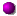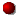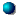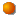# \$B%K!<%HWB@1(B

215P/NEAT###\$B2s5"5-O?(B\$BH/8+(B\$B=P8=(B\$B4QB,\$5\$l\$J\$+\$C\$?2s5"(B\$BH/8+A0\$N=P8=(B\$BH/8+A0\$N4QB,\$5\$l\$J\$+\$C\$?2s5"(B\$B>-Mh\$N2s5"(B215P 2019/11/18215P/2009 B5 2010/06/08215P/2002 O8 2002/05/08215P 1994/04/06##### 1994\$BG/\$N=P8=\$O(BMaik Meyer\$B;a\$N;XE&\$K\$h\$k\$b\$N(B(NK 1853)\$B!#(B 1994\$BG/6aF|E@DL2aF|\$O:4F#M55W;a\$N7W;;\$K\$h\$k\$b\$N!#(B